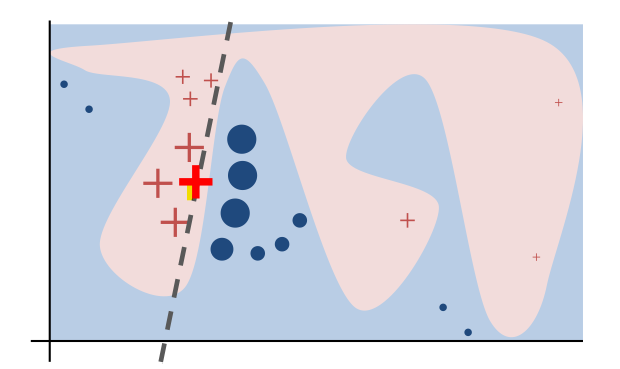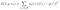# Immediately understand LIME for ML Model Explanation Part 1. Intuition Building

Building up intuition for using LIME to interpret machine learning models

Part 1. Intuition Building

Part 2. LIME for Image and Text Model Interpretation

LIME means Local interpretable model-agnostic explanations, which is a method to explain black box machine learning models by using local surrogate models. LIME’s explanation is for per instance, any is model-agnostic.

Here using Surrogate model means use a different interpretable model to approximate original black box model, then use this interpret-friendly model to explain the black box model prediction. The surrogate models can be linear regression, decision tree etc, which are relatively easier to interpret.

Local Surrogate model means instead of globally(for all observations) approximating the black box model, we just focus on approximating one observation’s behaviors in the black box, and only explain this observation’s behaviors via the surrogate model.

In the below example, red and blue area have very non-linear classification boundary, but for RED PLUS observation, we can use the simple regression line to explain its classification, and this is the general idea of LIME.Source from “Why Should I Trust You?”Explaining the Predictions of Any Classifier

# LIME Intuition Demystified

Given a trained model f(x1, x2, x3, , , xd) with d features and the training dataset is X which is a tabular dataset.

Below are LIME running steps to achieve explanation on instance x

Step 1. Create a new synthetic dataset Z’, Z’ has same feature schema as model training dataset X. But for each observation in Z’, we randomly set each feature value as 0 or 1, for example one observation in Z’ could be (x1=0, x2=1, x3=1, x4=0 , , , xd=1), feature value equal 0 or 1 is randomly set.

Step 2. Mapping Z’ to Z. The mapping is to mapping Z’ feature value 1 or 0 to the values which can be inputted into model f. The mapping rules are as following:

a. For categorical feature, 1 maps to corresponding feature value in instance y, 0 maps to any one different feature value from instance x based on corresponding feature’s distribution in training dataset X.

b. For numeric feature, 1 still maps to corresponding feature value in instance x, 0 mapping is sampling a value from a Normal(0,1) and doing the inverse operation(reverse standardized) of mean-centering and scaling, according to the means and std of corresponding feature in the training data X

Step 3. Predict the outcome of Z using the trained model, output is f(Z)

Step 4. Calculate weight for each observation in Z

In the weight formula, D is the distance between instance x and observations in Z, δ is a constant we can tuning.

From the formula we can see, Z observation which has larger distance to x will get smaller weight, the smaller distance will get larger weight, or in other words, the instance x’closer neighbors will get larger weight than remote neighbors.

Step 5. Now we can start to build the below linear regression, the regression independent variable is Z’, target variable is f(Z) which is known values.

also LIME introduces the below weighted least squares error as loss function for resolve the regression g(z’), the purpose for adding weight is we want regression g(z’ ) predicts more accurate on instance x’ s closer neighbors than remote neighbors, because we focus on instance x.Source from “Why Should I Trust You?”Explaining the Predictions of Any Classifier

So we have regression training data, target data and loss function, we can fix the regression.

Step 6. One more things LIME propose to resolve regression g(z’ ) is feature reduction. One of suggested way is to add LASSO(L1) regularization to the loss function, start from a very large regularization parameter λ which drive all feature coefficients towards 0, then slowly decrease λ, until there are Best-K feature coefficients not 0, then we will use the Best-K features to explain the regression.

# LIME Example

Firstly, we need install LIME python library by the following command

`pip install lime`

The example model is a trained RandomForestRegressor , the source data is Boston Housing price from Kaggle.

`import numpy as npimport pandas as pdimport limeimport lime.lime_tabularfrom sklearn.datasets import load_bostonfrom sklearn.ensemble import RandomForestRegressorfrom sklearn.model_selection import train_test_splitfrom sklearn import metricsboston = load_boston()X = pd.DataFrame(boston.data, columns=boston.feature_names)y = boston.targetX_train, X_test, y_train, y_test = train_test_split(X, y, test_size=0.20, random_state=22)model = RandomForestRegressor(n_estimators=1000)model.fit(X_train, y_train)y_pred = model.predict(X_test)print('RMSE:', np.sqrt(metrics.mean_squared_error(y_test, y_pred)))print('R-Squared:', metrics.r2_score(y_test, y_pred))#RMSE: 3.959655514886183#R-Squared: 0.8231724590161542`

From LIME intuition, we understand LIME use different way to mapping categorical and numeric feature, so we need find out categorical features from training dataset, and pass the categorical features information to LIME explainer.

Below code check how many unique values in each feature, if the number of unique value is equal or less then 10, then we think the feature is categorical feature.

`categorical_features = np.argwhere(    np.array([len(set(boston.data[:,x])) for x in range(boston.data.shape)]) <= 10).flatten()`

Also we choose an instance from test set, and use LIME explainer to explain the instance’s prediction, the chosen instance as below

`X_test.iloc[10,:]`

Next is to create LIME explainer and run the explanation on the chosen instance, also we reduce the feature size to 5.

Please refer to https://lime-ml.readthedocs.io/en/latest/lime.html#module-lime.lime_tabular for method details.

`explainer = lime.lime_tabular.LimeTabularExplainer(    train,     feature_names=boston.feature_names,     class_names=['price'],     categorical_features=categorical_features,     verbose=True,     mode='regression',    discretize_continuous=False)#Intercept 24.1171706493745#Prediction_local [22.36560785]#Right(Model Prediction): 19.744900000000076`

Instance explanation

`exp = explainer.explain_instance(    X_test.iloc[10,:],     model.predict,     num_features=5)exp.show_in_notebook(show_table=True)`
`exp.as_list()# local surrogate regression coefficients#[('LSTAT', -4.550192483817742),# ('RM', 2.6441938803314087),# ('DIS', -1.9802639380327312),# ('TAX', -0.3144135103939565),# ('PTRATIO', -0.2682944259385174)]`

19.74 is RandomForestRegressor model prediction, 22.37 is local surrogate regression output, the two values are close(we can tuning the weight and explainer parameters to make these two prediction are closer). So we can use the local surrogate regression coefficients to explain each feature contribution to RandomForestRegressor model output on target instance.

# Summary

I hope this story can help you quickly build up the intuition of LIME, and the take away point is LIME is using an interpretable surrogate model to locally approximates the prediction of black box model on a target instance. And explain the target instance’s prediction via the surrogate model. In next story we will explore how LIME be used for Text and Image data Part 2.

# REFERENCES

1. Interpretable Machine Learning: https://christophm.github.io/interpretable-ml-book/shap.html
2. “Why Should I Trust You?”
Explaining the Predictions of Any Classifier : https://arxiv.org/pdf/1602.04938.pdf
3. The Science Behind InterpretML: LIME https://www.youtube.com/watch?v=g2WtL45-PFQ&feature=emb_rel_end
4. Interpretable Machine Learning Using LIME Framework — Kasia Kulma (PhD) https://www.youtube.com/watch?v=CY3t11vuuOM&t=888s
5. Understanding how LIME explains predictions https://towardsdatascience.com/understanding-how-lime-explains-predictions-d404e5d1829c
6. https://github.com/marcotcr/lime
7. https://lime-ml.readthedocs.io/en/latest/index.html

Data Scientist & Engineer from Sydney

## More from Summer Hu

Data Scientist & Engineer from Sydney